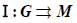期刊菜单

Symplectic Reduction for Cotangent Groupoids
DOI: 10.12677/PM.2021.113043, PDF, HTML, XML, 下载: 101  浏览: 873

Abstract: Given a Lie groupoidand I-space N, this paper considers symplectic groupoid actions of the cotangent groupoidon the cotangent bundle TN. Meanwhile, this reduction is investigated concretely.

1. 引言

2. 余切群胚

1. 对任意 $\left(h,g\right)\in {G}_{2}$，有 $\alpha \left(hg\right)=\alpha \left(g\right)$$\beta \left(hg\right)=\beta \left(h\right)$

2. 对任意 $g,h,k\in G$，有 $\left(gh\right)k=g\left(hk\right)$，其中 $\alpha \left(g\right)=\beta \left(h\right)$$\alpha \left(h\right)=\beta \left(k\right)$

3. 对任意 $x\in M$$\alpha \left({1}_{x}\right)=\beta \left({1}_{x}\right)=x$

4. 对任意 $g\in G$，有 $g{1}_{\alpha g}=g,{1}_{\beta g}g=g$

5. 对任意 $g\in G$，G中存在逆元 ${g}^{-1}$，满足 $\alpha \left({g}^{-1}\right)=\beta \left(g\right)$$\beta \left({g}^{-1}\right)=\alpha \left(g\right)$${g}^{-1}g={1}_{\alpha g}$$g{g}^{-1}={1}_{\beta g}$

① 定义 $\stackrel{˜}{\alpha }:{T}^{\ast }G\to {A}^{\ast }G,\varphi ↦\stackrel{˜}{\alpha }\left(\varphi \right)$，使得对 $\forall X\in {A}_{\alpha g}G$，有 $〈\stackrel{˜}{\alpha }\left(\varphi \right),X〉=〈\varphi ,T\left({L}_{g}\right)\left(X-T\left(1\right)aX\right)〉$。其中 $a={a}_{G}:AG\to TM$ 为向量丛间的丛映射使得对 $\forall x\in M$${a}_{x}:{A}_{x}G\to {T}_{x}M$$X↦d\beta |{}_{{1}_{x}}\left(X\right)$，这里

$X\in {T}_{{1}_{x}}G$ 满足 ${d\alpha |}_{{}_{{1}_{x}}}\left(X\right)=0$

② 定义 $\stackrel{˜}{\beta }:{T}^{\ast }G\to {A}^{\ast }G,\varphi ↦\stackrel{˜}{\beta }\left(\varphi \right)$，使得对 $\forall Y\in {A}_{\beta g}G$，有 $〈\stackrel{˜}{\beta }\left(\varphi \right),Y〉=〈\varphi ,T\left({R}_{g}\right)Y〉$

③ 定义乘法运算 $·:{T}^{\ast }G×{T}^{\ast }G\to {T}^{\ast }G$$\left(\varphi ,\psi \right)↦\varphi ·\psi$。其中 $\varphi \in {T}_{g}^{\ast }G,\psi \in {T}_{h}^{\ast }G$ 满足 $\stackrel{˜}{\alpha }\left(\varphi \right)=\stackrel{˜}{\beta }\left(\psi \right)$。这里 $X\in {T}_{g}G$$Y\in {T}_{h}G$ 满足 $T\left(\alpha \right)X=T\left(\beta \right)Y$ 使得 $〈\varphi ·\psi ,X·Y〉=〈\varphi ,X〉+〈\psi ,Y〉$

④ 定义单位映射 $\stackrel{˜}{1}:{A}^{\ast }G\to {T}^{\ast }G$，使得对 $\forall \phi \in {A}_{m}^{\ast }G,\xi \in {T}_{1m}G$，有 $〈{\stackrel{˜}{1}}_{\phi },\xi 〉=〈\phi ,\xi -T\left(1\right)T\left(\alpha \right)\xi 〉$

⑤ 定义逆运算： ${T}^{\ast }G\to {T}^{\ast }G,\varphi ↦{\varphi }^{-1}$。使得对 $\forall X\in {T}_{g}G$$〈{\varphi }^{-1},{X}^{-1}〉=-〈\varphi ,X〉$ (其中

$\varphi \in {T}_{g}^{\ast }G,{X}^{-1}\in {T}_{{g}^{-1}}G$ 为X在逆运算 $G\to G$ 的诱导切映射 ${T}_{g}G\to {T}_{{g}^{-1}}G$ 下的像)。

① 任取 $\varphi \in {T}_{g}^{\ast }G,\psi \in {T}_{h}^{\ast }G$，使得 $\stackrel{˜}{\alpha }\left(\varphi \right)=\stackrel{˜}{\beta }\left(\psi \right)$，则 $\varphi ·\psi \in {T}_{gh}^{\ast }G$。需说明 $\stackrel{˜}{\alpha }\left(\varphi ·\psi \right)=\stackrel{˜}{\alpha }\left(\psi \right)$$\stackrel{˜}{\beta }\left(\varphi ·\psi \right)=\stackrel{˜}{\beta }\left(\varphi \right)$

$\begin{array}{c}〈\stackrel{˜}{\alpha }\left(\varphi ·\psi \right),X〉\\ =〈\varphi ·\psi ,T\left({L}_{gh}\right)\left(X-T\left(1\right)aX\right)〉=〈\varphi ·\psi ,0·T\left({L}_{h}\right)\left(X-T\left(1\right)aX\right)〉\\ =〈\varphi ,0〉+〈\psi ,T\left({L}_{h}\right)\left(X-T\left(1\right)aX\right)〉=〈\stackrel{˜}{\alpha }\left(\psi \right),X〉\end{array}$

$\forall Y\in {A}_{\beta \left(gh\right)}G={A}_{\beta \left(g\right)}G$，有

$\begin{array}{c}〈\stackrel{˜}{\beta }\left(\varphi ·\psi \right),Y〉=〈\varphi ·\psi ,T\left({R}_{gh}\right)\left(Y\right)〉=〈\varphi ·\psi ,T\left({R}_{g}\right)\left(Y\right)·0〉\\ =〈\varphi ,T\left({R}_{g}\right)\left(Y\right)〉+〈\psi ,0〉=〈\stackrel{˜}{\beta }\left(\varphi \right),Y〉\end{array}$

② 任取 ${\varphi }_{1}\in {T}_{f}^{\ast }G,{\varphi }_{2}\in {T}_{g}^{\ast }G,{\varphi }_{3}\in {T}_{h}^{\ast }G$，且满足 $\stackrel{˜}{\alpha }\left({\varphi }_{1}\right)=\stackrel{˜}{\beta }\left({\varphi }_{2}\right),\stackrel{˜}{\alpha }\left({\varphi }_{2}\right)=\stackrel{˜}{\beta }\left({\varphi }_{3}\right)$。需说明 ${\varphi }_{1}·\left({\varphi }_{2}·{\varphi }_{3}\right)=\left({\varphi }_{1}·{\varphi }_{2}\right)·{\varphi }_{3}$

③ 任取 $\phi \in {A}_{m}^{\ast }G$，需说明 $\stackrel{˜}{\alpha }\left({1}_{\phi }\right)=\stackrel{˜}{\beta }\left({1}_{\phi }\right)=\phi$

$\begin{array}{c}〈\stackrel{˜}{\alpha }\left({\stackrel{˜}{1}}_{\phi }\right),X〉=〈{\stackrel{˜}{1}}_{\phi },T\left({L}_{1m}\right)\left(X-T\left(1\right)aX\right)〉=〈{\stackrel{˜}{1}}_{\phi },\left(X-T\left(1\right)aX\right)〉\\ =〈\phi ,\left(X-T\left(1\right)aX\right)-T\left(1\right)T\left(\alpha \right)\left(X-T\left(1\right)aX\right)〉\\ =〈\phi ,X〉\end{array}$

④ 任取 $\varphi \in {T}_{g}^{\ast }G$，需说明 $\varphi ·{\stackrel{˜}{1}}_{\stackrel{˜}{\alpha }\left(\varphi \right)}=\varphi$${\stackrel{˜}{1}}_{\stackrel{˜}{\beta }\left(\varphi \right)}·\varphi =\varphi$

$\begin{array}{c}〈\varphi ·{\stackrel{˜}{1}}_{\stackrel{˜}{\alpha }\left(\varphi \right)},X·Y〉=〈\varphi ,X〉+〈{\stackrel{˜}{1}}_{\stackrel{˜}{\alpha }\left(\varphi \right)},Y〉=〈\varphi ,X〉+〈\stackrel{˜}{\alpha }\left(\varphi \right),Y-T\left(1\right)T\left(\alpha \right)Y〉\\ =〈\varphi ,X〉+〈\varphi ,T\left({L}_{g}\right)\left[\left(Y-T\left(1\right)T\left(\alpha \right)Y\right)-T\left(1\right)a\left(Y-T\left(1\right)T\left(\alpha \right)Y\right)\right]〉\\ =〈\varphi ,X〉+〈\varphi ,T\left({L}_{g}\right)\left[\left(Y-T\left(1\right)T\left(\alpha \right)Y\right)-T\left(1\right)T\left(\beta \right)\left(Y-T\left(1\right)T\left(\alpha \right)Y\right)\right]〉\\ =〈\varphi ,X〉+〈\varphi ,T\left({L}_{g}\right)\left(Y-T\left(1\right)T\left(\beta \right)Y\right)〉\\ =〈\varphi ,X+T\left({L}_{g}\right)\left(Y-T\left(1\right)T\left(\beta \right)Y\right)〉=〈\varphi ,X·Y〉\end{array}$

$\begin{array}{c}〈{\stackrel{˜}{1}}_{\stackrel{˜}{\beta }\left(\varphi \right)}·\varphi ,Z·X〉=〈{\stackrel{˜}{1}}_{\stackrel{˜}{\beta }\left(\varphi \right)},Z〉+〈\varphi ,X〉=〈\stackrel{˜}{\beta }\left(\varphi \right),Z-T\left(1\right)T\left(\alpha \right)Z〉+〈\varphi ,X〉\\ =〈\varphi ,T\left({R}_{g}\right)\left(Z-T\left(1\right)T\left(\alpha \right)Z\right)〉+〈\varphi ,X〉\\ =〈\varphi ,T\left({R}_{g}\right)\left(Z-T\left(1\right)T\left(\alpha \right)Z\right)+X〉=〈\varphi ,Z·X〉\end{array}$

⑤ 任取 $\varphi \in {T}_{g}^{\ast }G$，需说明 $\stackrel{˜}{\alpha }\left({\varphi }^{-1}\right)=\stackrel{˜}{\beta }\left(\varphi \right)$$\stackrel{˜}{\beta }\left({\varphi }^{-1}\right)=\stackrel{˜}{\alpha }\left(\varphi \right)$$\varphi ·{\varphi }^{-1}={\stackrel{˜}{1}}_{\stackrel{˜}{\beta }\left(\varphi \right)}$${\varphi }^{-1}·\varphi ={\stackrel{˜}{1}}_{\stackrel{˜}{\alpha }\left(\varphi \right)}$

$\begin{array}{c}〈\stackrel{˜}{\alpha }\left({\varphi }^{-1}\right),X〉=〈{\varphi }^{-1},T\left({L}_{{g}^{-1}}\right)\left(X-T\left(1\right)aX\right)〉=〈{\varphi }^{-1},T\left({L}_{{g}^{-1}}\right)\left(-T\left(i\right)X\right)〉\\ =〈{\varphi }^{-1},-T\left(i\right)T\left({R}_{g}\right)X〉=〈\varphi ,T\left({R}_{g}\right)X〉=〈\stackrel{˜}{\beta }\left(\varphi \right),X〉\end{array}$

$T\left(1\right)\left(w\right)+W$，其中 $w\in {T}_{\beta g}M,W\in {A}_{\beta g}G$

$〈\varphi ·{\varphi }^{-1},T\left(1\right)w〉=〈\varphi ·{\varphi }^{-1},Y·{Y}^{-1}〉=〈\varphi ,Y〉+〈{\varphi }^{-1},{Y}^{-1}〉=〈\varphi ,Y〉-〈\varphi ,Y〉=0$

$〈{\stackrel{˜}{1}}_{\stackrel{˜}{\beta }\left(\varphi \right)},T\left(1\right)w〉=〈{\stackrel{˜}{1}}_{\stackrel{˜}{\beta }\left(\varphi \right)},T\left(1\right)T\left(\alpha \right)X〉=0$，故 $〈\varphi ·{\varphi }^{-1},T\left(1\right)w〉=〈{\stackrel{˜}{1}}_{\stackrel{˜}{\beta }\left(\varphi \right)},T\left(1\right)w〉$

${\stackrel{˜}{0}}_{{g}^{-1}}\in {T}_{{g}^{-1}}G$ 为切空间 ${T}_{{g}^{-1}}G$ 中的零向量，则

$W=\left(T\left({R}_{g}\right)W\right)·{\stackrel{˜}{0}}_{{g}^{-1}}$，(*)

$〈\varphi ·{\varphi }^{-1},W〉=〈\varphi ·{\varphi }^{-1},\left(T\left({R}_{g}\right)\right)W·{\stackrel{˜}{0}}_{{g}^{-1}}〉=〈\varphi ,\left(T\left({R}_{g}\right)\right)W〉+〈{\varphi }^{-1},{\stackrel{˜}{0}}_{{g}^{-1}}〉=〈\varphi ,T\left({R}_{g}\right)W〉=〈\stackrel{˜}{\beta }\left(\varphi \right),W〉=〈{1}_{\stackrel{˜}{\beta }\left(\varphi \right)},W〉$

$\gamma \left(0\right)={1}_{y},\alpha \left(\gamma \left(t\right)\right)=y$${\frac{\text{d}}{\text{d}t}|}_{t=0}\gamma \left(t\right)=W$。从而 $T\left({R}_{g}\right)W={\frac{\text{d}}{\text{d}t}|}_{t=0}{R}_{g}\left(\gamma \left(t\right)\right)={\frac{\text{d}}{\text{d}t}|}_{t=0}\gamma \left(t\right)g$ 从而

$T\left({R}_{g}\right)W·{\stackrel{˜}{0}}_{{g}^{-1}}={\frac{\text{d}}{\text{d}t}|}_{t=0}k\left(\gamma \left(t\right)g,{g}^{-1}\right)={\frac{\text{d}}{\text{d}t}|}_{t=0}\gamma \left(t\right)=W$

(2) 证明 $G=\left\{\left(g,\varphi \right),\left(h,\psi \right),\left(gh,\varphi ·\psi \right)\in {T}^{\ast }G×{T}^{\ast }G×{T}^{\ast }G|\stackrel{˜}{\alpha }\left(\varphi \right)=\stackrel{˜}{\beta }\left(\psi \right)\right\}$ 是辛流形 $\left({T}^{\ast }G,{w}_{0}\right)×\left({T}^{\ast }G,{w}_{0}\right)×\left({T}^{\ast }G,-{w}_{0}\right)$ 的拉格朗日子流形，则 ${T}^{\ast }G⇉{A}^{\ast }G$ 为辛群胚。

$\left\{\left(\varphi ,\psi ,-\varphi ·\psi \right)\in {T}_{g}^{\ast }G×{T}_{h}^{\ast }G×{T}_{gh}^{\ast }G|\stackrel{˜}{\alpha }\left(\varphi \right)=\stackrel{˜}{\beta }\left(\psi \right)\right\}$

3. 主要结果及证明

$G⇉M$ 是一个李群胚，N是一个 ${C}^{\infty }$ 流形，并且 $J:N\to M$${C}^{\infty }$ 映射。

(1) $J\left(gn\right)=\beta \left(g\right)$

(2) $\left(gh\right)n=g\left(hn\right)$

(3) $1\left(J\left(n\right)\right)n=n$

${J}_{q}^{\ast }{\varphi }_{q}\in {\left({A}^{\ast }G\right)}_{J\left(q\right)}$ 使得对 $\forall X\in {\left(AG\right)}_{J\left(q\right)}={T}_{{1}_{J\left(q\right)}}{\alpha }^{-1}\left(J\left(q\right)\right)$$〈{J}_{q}^{\ast }{\varphi }_{q},X〉=〈{\varphi }_{q},{X}^{#}\left(q\right)〉$ (其中 ${X}^{#}\left(q\right)\in {T}_{J\left(q\right)}N$ 为由X诱导的切向量：任取 ${\alpha }^{-1}\left(J\left(q\right)\right)\subset G$ 中通过 ${1}_{J\left(q\right)}$${C}^{\infty }$ 曲线 $\gamma :\left(-\epsilon ,\epsilon \right)\to {\alpha }^{-1}\left(J\left(q\right)\right)$$\gamma \left(0\right)={1}_{J\left(q\right)}$ 使得 ${\frac{\text{d}\gamma \left(t\right)}{\text{d}t}|}_{t=0}=X$，则 ${X}^{#}\left(q\right):={\frac{\text{d}}{\text{d}t}|}_{t=0}\gamma \left(t\right)q\in {T}_{q}N$ )。定义 ${J}^{\ast }:{T}^{\ast }N\to {A}^{\ast }G$$\left(q,{\varphi }_{q}\right)↦\left(J\left(q\right),{J}_{q}^{\ast }{\varphi }_{q}\right)$，显然 ${J}^{\ast }$ 是光滑的丛映射且使得图表 $\begin{array}{l}\text{\hspace{0.17em}}{T}^{\ast }N\to {A}^{\ast }G\\ {}_{{\pi }_{N}}↓\text{}{↓}_{{\pi }_{M}}\\ \text{}N\text{\hspace{0.17em}}\text{ }\text{ }\to \text{ }\text{\hspace{0.17em}}M\end{array}$ 可交换，其中 ${\pi }_{N},{\pi }_{M}$ 均为丛投影映射。

${0}_{m}\in {A}^{\ast }G$ 为clean值使得 ${\left({J}^{\ast }\right)}^{-1}\left({0}_{m}\right)/{G}_{m}$${C}^{\infty }$ 流形，且 ${\pi }_{0}:{\left({J}^{\ast }\right)}^{-1}\left({0}_{m}\right)\to {\left({J}^{\ast }\right)}^{-1}\left({0}_{m}\right)/{G}_{m}$ 为浸没映射，假

${\left({J}^{\ast }\right)}^{-1}\left({0}_{m}\right)/{G}_{m}$ 辛微分同胚于余切丛 ${T}^{\ast }\left({J}^{-1}\left(m\right)/{G}_{m}\right)$

$〈{\stackrel{¯}{\phi }}_{0}\left(g\cdot {\varphi }_{q}\right),{T}_{q}{\pi }_{J}\left({v}_{q}\right)〉=〈{\stackrel{¯}{\phi }}_{0}\left(g\cdot {\varphi }_{q}\right),{T}_{g\cdot q}{\pi }_{J}\left(g\cdot {v}_{q}\right)〉=〈g\cdot {\varphi }_{q},g\cdot {v}_{q}〉=〈{\varphi }_{q},{v}_{q}〉=〈{\stackrel{¯}{\phi }}_{0}\left({\varphi }_{q}\right),{T}_{q}{\pi }_{J}\left({v}_{q}\right)〉$，所以对于

$〈{\varphi }_{q},{v}_{q}〉:=〈{\Gamma }_{\left[q\right]},{T}_{q}{\pi }_{J}\left({v}_{q}\right)〉$，那么 ${\stackrel{¯}{\phi }}_{0}\left({\varphi }_{q}\right)={\Gamma }_{\left[q\right]}$

${\pi }_{0}:{\left({J}^{\ast }\right)}^{-1}\left({0}_{m}\right)\to {\left({J}^{\ast }\right)}^{-1}\left({0}_{m}\right)/{G}_{m}$ )。

$g\in {G}_{m}$ 使得 $q\prime =g\cdot q$。因为 ${J}^{\ast }\left(g\cdot {\varphi }_{q}\right)=A{d}_{{g}^{-1}}^{\ast }{J}^{\ast }\left({\varphi }_{q}\right)=0$ 可知 $g\cdot {\alpha }_{q},{\alpha }_{q\prime }\in {\left({J}^{\ast }\right)}^{-1}\left({0}_{m}\right)\cap {T}_{q\prime }^{\ast }N$。由于 ${\phi }_{0}$${G}_{m}$ -

${\pi }_{{J}^{-1}\left(m\right)/{G}_{m}}:{T}^{\ast }\left({J}^{-1}\left(m\right)/{G}_{m}\right)\to {J}^{-1}\left(m\right)/{G}_{m}$ 为余切丛投影，显然有 ${\pi }_{{J}^{-1}\left(m\right)/{G}_{m}}\circ {\stackrel{¯}{\phi }}_{0}={\pi }_{J}\circ {\pi }_{{J}^{-1}\left(m\right)}\circ {i}_{0}$。取

${\varphi }_{q}\in {\left({J}^{\ast }\right)}^{-1}\left({0}_{m}\right),v\in {T}_{{\varphi }_{q}}{\left({J}^{\ast }\right)}^{-1}\left({0}_{m}\right)$，则

$\begin{array}{c}〈\left({\pi }_{0}^{\ast }{\phi }_{0}^{\ast }{\theta }_{can}\right)\left({\varphi }_{q}\right),v〉=〈\left({\stackrel{¯}{\phi }}_{0}^{\ast }{\theta }_{can}\right){\varphi }_{q},v〉=〈{\theta }_{can}\left({\stackrel{¯}{\phi }}_{0}\left({\varphi }_{q}\right)\right),{T}_{{\varphi }_{q}}{\stackrel{¯}{\phi }}_{0}\left(v\right)〉\\ =〈{\stackrel{¯}{\phi }}_{0}\left({\varphi }_{q}\right),{T}_{{\stackrel{¯}{\phi }}_{0}\left({\varphi }_{q}\right)}{\pi }_{{\left({J}^{\ast }\right)}^{-1}\left({0}_{m}\right)}\left({T}_{{\varphi }_{q}}{\stackrel{¯}{\phi }}_{0}\left(v\right)\right)〉\\ =〈{\stackrel{¯}{\phi }}_{0}\left({\varphi }_{q}\right),{T}_{{\varphi }_{q}}\left({\pi }_{{\left({J}^{\ast }\right)}^{-1}\left({0}_{m}\right)}\circ {\stackrel{¯}{\phi }}_{0}\right)\left(v\right)〉\\ =〈{\stackrel{¯}{\phi }}_{0}\left({\varphi }_{q}\right),{T}_{{\varphi }_{q}}\left({\pi }_{J}\circ {\pi }_{{J}^{-1}\left(m\right)}\circ {i}_{0}\right)\left(v\right)〉\\ =〈{\stackrel{¯}{\phi }}_{0}\left({\varphi }_{q}\right),{T}_{q}{\pi }_{J}\left({T}_{{\varphi }_{q}}{\pi }_{{J}^{-1}\left(m\right)}\left(v\right)\right)〉\\ =〈{\varphi }_{q},{T}_{{\varphi }_{q}}{\pi }_{{J}^{-1}\left(m\right)}\left(v\right)〉=〈{i}_{0}^{\ast }\Theta \left({\varphi }_{q}\right),v〉\end{array}$

${\pi }_{0}^{\ast }{\phi }_{0}^{\ast }{\theta }_{can}={i}_{0}^{\ast }{\Theta }_{can}$。因此 ${\pi }_{0}^{\ast }{\phi }_{0}^{\ast }{w}_{can}={i}_{0}^{\ast }{\Omega }_{can}$，其中 ${w}_{can},{\Omega }_{can}$ 分别为 ${T}^{\ast }\left({J}^{-1}\left(m\right)/{G}_{m}\right),{T}^{\ast }\left({J}^{-1}\left(m\right)\right)$ 的典范辛形式。由辛约化定理可知， ${\phi }_{0}^{\ast }{w}_{can}={\Omega }_{0}$，其中 ${\Omega }_{0}$${\left({J}^{\ast }\right)}^{-1}\left({0}_{m}\right)/{G}_{m}$ 的约化辛形式。

$\mathrm{dim}{\left({J}^{\ast }\right)}^{-1}\left({0}_{m}\right)/{G}_{m}=2\mathrm{dim}{J}^{-1}\left(m\right)-2\mathrm{dim}{G}_{m}=\mathrm{dim}{T}^{\ast }\left({J}^{-1}\left(m\right)/{G}_{m}\right)$

${\phi }_{0}$ 为局部微分同胚映射。又由于 ${\phi }_{0}$ 为双射，从而 ${\phi }_{0}$ 为微分同胚映射。

  Arnold, V. (1966) Sur la gèométrie diffèrentielle des groupes de Lie de dimension infinie et ses applications à l’hydrodynamique des fluides parfaits. Annales de l’Institut Fourier, 16, 319-361. https://doi.org/10.5802/aif.233  Smale, S. (1970) Topology and Mechanics. I. Inventiones Mathematicae, 10, 305-331. https://doi.org/10.1007/BF01418778  Meyer, K.R. (1973) Symmetries and Integrals in Mathematics. Dy-namical Systems, Academic Press, New York, 259-272. https://doi.org/10.1016/B978-0-12-550350-1.50025-4  Marsden, J. and Weinstein, A. (1974) Reduction of Symplectic Manifolds with Symmetry. Reports on Mathematical Physics, 5, 121-130. https://doi.org/10.1016/0034-4877(74)90021-4  Marsden, J.E., Misiolek, G., Ortega, J.P., et al. (2007) Ham-iltonian Reduction by Stages. Springer, New York, 1-64.  Mackenzie, K.C.H. (2005) General Theory of Lie Groupoids and Lie Algebroids. Cambridge University Press, New York. https://doi.org/10.1017/CBO9781107325883  da Silva, A.C. (2008) Lectures on Symplectic Geometry. Springer, New York, 7-22. https://doi.org/10.1007/978-3-540-45330-7  Mikami, K. and Weinstein, A. (1988) Moments and Reduction for Symplectic Groupoids. Publications of the Research Institute for Mathematical Sciences, 24, 121-140. https://doi.org/10.2977/prims/1195175328